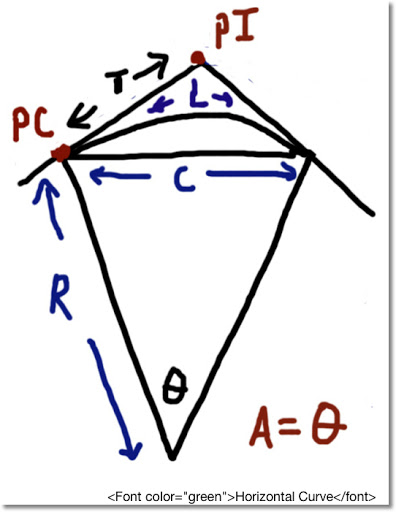## Sunday, May 19, 2013

### HP35S: Horizontal Curve - Finding Radius, Chord Length, and Arc LengthHP 35S: Horizontal Curve
Original: HP 33S Surveying Applications, Hewlett Packard, March 1978, pg. 46

Calculator
HP 35S

Input
(see diagram above)

T = Tangent Distance (length of segment from P.C. (Point of Curvature) to P.I. (Point of Tangent Intersection))
A = Central curve in degrees, minutes, seconds

This program prompts for tangent length and central angle

Output

The program gives the following results:
1. Radius of the horizontal curve (R)
2. Press R/S to get the Chord length (C)
3. Press R/S once more to get the arc length of the horizontal curve (L)

The program does not store any results.

Formulas
R = T × (tan(A/2))⁻¹
C = 2 × R × sin(A/2)
L = R × A in radians

Where
T = tangent distance
A = central angle
C = Chord Length
L = Arc Length

Example
Tangent Length: 172.45
Central Angle: 40°22'13" (enter as 40.2213)

Results:
Chord Length: 323.7172
Arc Length: 330.51163

Program
V001 LBL V
V002 DEG
V003 INPUT T
V004 INPUT A
V005 HMS→\\ sometimes named ->H
V006 STO A
V007 2
V008 ÷
V009 TAN
V010 1/x
V011 ×
V013 ENTER
V014 ENTER
V015 2
V016 ×
V017 RCL A
V018 2
V019 ÷
V020 SIN
V021 ×
V022 R/S \\ display Chord Length
V023 x<>y
V024 RCL A
V026 ×
V027 RTN \\ display Arc Length

If you don't have the ->RAD function, you can substitute the following steps:
π, ×, 180, ÷

This blog is property of Edward Shore. 2013

1.Thank you for sharing your thoughts. I truly appreciate your effort in sharing this informative articles. Very impressive and quite interesting for the readers. Good job.

Sol
www.gofastek.com

2.Hi, very smooth programming. Just be careful that you don't run some misc. calc. after it outputs the Chord, because the Stack will not be aligned for the third and last calc.

3.Hi, very smooth programming. Just be careful that you don't run some misc. calc. after it outputs the Chord, because the Stack will not be aligned for the third and last calc.

### Σ(1 / (a^n)) from n=1 to m

Σ(1 / (a^n)) from n=1 to m This blog entry covers the sum of the series: Σ[1 / (a^n), n=1 to m] with n and m positive integers Specific Cas...